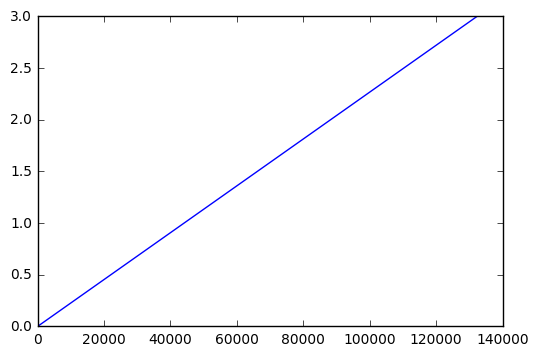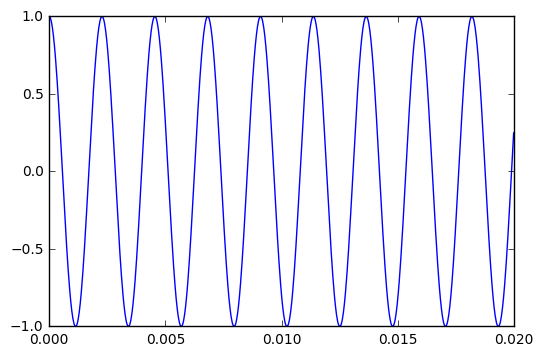# Preamble: Dependencies¶

NumPy and Matplotlib are Python Libraries that are widely used in scientific computing and data visualisation.

In [ ]:
from numpy import *
from matplotlib.pyplot import *
%matplotlib inline


The audio package is a collection of Python modules that I have developped for this course:

• use audio.wave to read/write WAVE files,

• use audio.io to play/record sound data,

• use audio.bitstream to read/write binary data.

In :
import audio.io
import audio.wave
from audio.bitstream import BitStream


# Synthesis of Pure Tones¶

In :
df = 44100.0
dt = 1.0 / df
f = 440.0
T = 3.0

In :
t = r_[0.0:T:dt] # see also: arange, linspace, etc.
t[-1] # the last value (T) is excluded

Out:
2.9999773242630385
In :
plot(t)

Out:
[<matplotlib.lines.Line2D at 0x7faac804b450>]In :
A4 = cos(2*pi*f*t)

In :
audio.io.play(A4)

In :
ts = t[t<20.0/1000.0] # 20 ms
A4s = A4[t<20.0/1000.0]
plot(ts, A4s)

Out:
[<matplotlib.lines.Line2D at 0x7faac3016310>]In :
def make_tone(symbol):
number = int(symbol[1:])
f = 27.5 * 2 ** number
x = cos(2*pi*f*t)
audio.wave.write(x, symbol + ".wav")
return x


In :
A8 = make_tone("A8")
audio.io.play(A8)

In :
A = []
for i in range(0,11):
symbol = "A" + str(i)
A.append(make_tone(symbol))

In :
for i, sound in enumerate(A):
print "A" + str(i)
audio.io.play(sound)

A0
A1
A2
A3
A4
A5
A6
A7
A8
A9
A10

In :
27.5 * 2**10

Out:
28160.0
In :
audio.io.play(A)

In :
for sound in A:
print mean(sound*sound)

0.5
0.5
0.5
0.5
0.5
0.5
0.5
0.5
0.5
0.5
0.5

In :
def L(x):
return 96.0 + 10.0 * log10(mean(x*x))

In :
L(A)

Out:
92.989700043360187

In :
audio.io.play(A)

In :
audio.io.play(A, df=16000)


Wave format documentation: http://soundfile.sapp.org/doc/WaveFormat/

In :
raw = open("A4.wav").read() # raw is a 'str' (string)
print raw[:4], raw[8:12]

RIFF WAVE

In :
raw = open("A4.wav").read()
stream = BitStream(raw)

RIFF
WAVE

In :
raw = open("A4.wav").read()
stream = BitStream(raw)
print stream.read(uint16).newbyteorder() # 2-byte integer (little end.)
print stream.read(uint32).newbyteorder() # 4-byte integer (little end.)

1
44100


# Quantization and Signal-to-Noise Ratio¶

In :
A4q = audio.wave.read("A4.wav")
e = A4 - A4q
SNR = sqrt(mean(A4*A4) / mean(e * e))
print SNR

77863.1828913

In :
print 20.0 * log10(SNR) # SNR in dB

97.8266430567